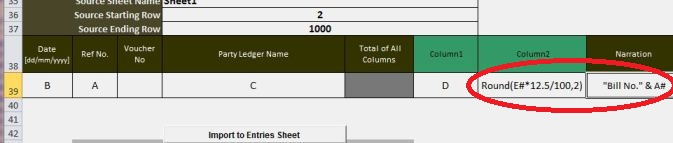# Excel to Tally : Map excel sheet with Microsoft Excel formulas on the fly...

You can import your excel data using Excel Formulas like a magic. You don't have to alter your existing excel sheet, just MAP your excel sheet columns with EA4 Templates & thats all. While Mapping your sheet with EA4 templates you can use Excel Formulas in column names to import calculated data.Following table is showing how formulas can be used with ease while importing entries to templates.

 Sr. Description Excel Formula EazyAUTO4 Template Formula 1 It is possible to import calculated columns =a1*12.5/100 a#*12.5/100 2 Value can be take from one or more column from source sheet. =(a1-b1+c1) *12.5/100 (a#-b#+c#) *12.5/100 3 Conditional value import from source sheet =if(a1>0,c1,d1) if(a#>0,c#,d#) 4 Formals can be used to get calculated string column ="Bill No." & d1 "Bill No." & d# 5 Rounding to 2 decimals =round(a1,2) round(a#,2) 6 Autofill column for blank cells using first used cell Possible using "Go to special" option with select "Blanks" command {a} 7 Calculate using absolute cell with relative address. It will add value of cell b100 to each cell. =a1+b100 a#+b\$100 8 Using offset formula to get value =Offset(a1,0,-1) Offset(a#,0,-1) 9 Using sum formula for absolute address =SUM("A1:D1") SUM("A1:D1") 10 Using sum formula for relative address =SUM("A1:D1") SUM("A#:D#") Above are some examples, but you can use ALL formulas available in excel Some point to remember while using formulas to import data from source sheet to EazyAUTO4 Template 1 Use "#" for relative row numbers like a#,b#... 2 Use absolute cell address like A10,B25... to calculate using absolute address... 3 DO NOT start WITH '=' sign
CompanyImpression Systems & Software Pvt. Ltd.
Email : mail@eazy.co.in
Call : +91-9011031113

© 2021 Impression Systems & Software Pvt. Ltd.